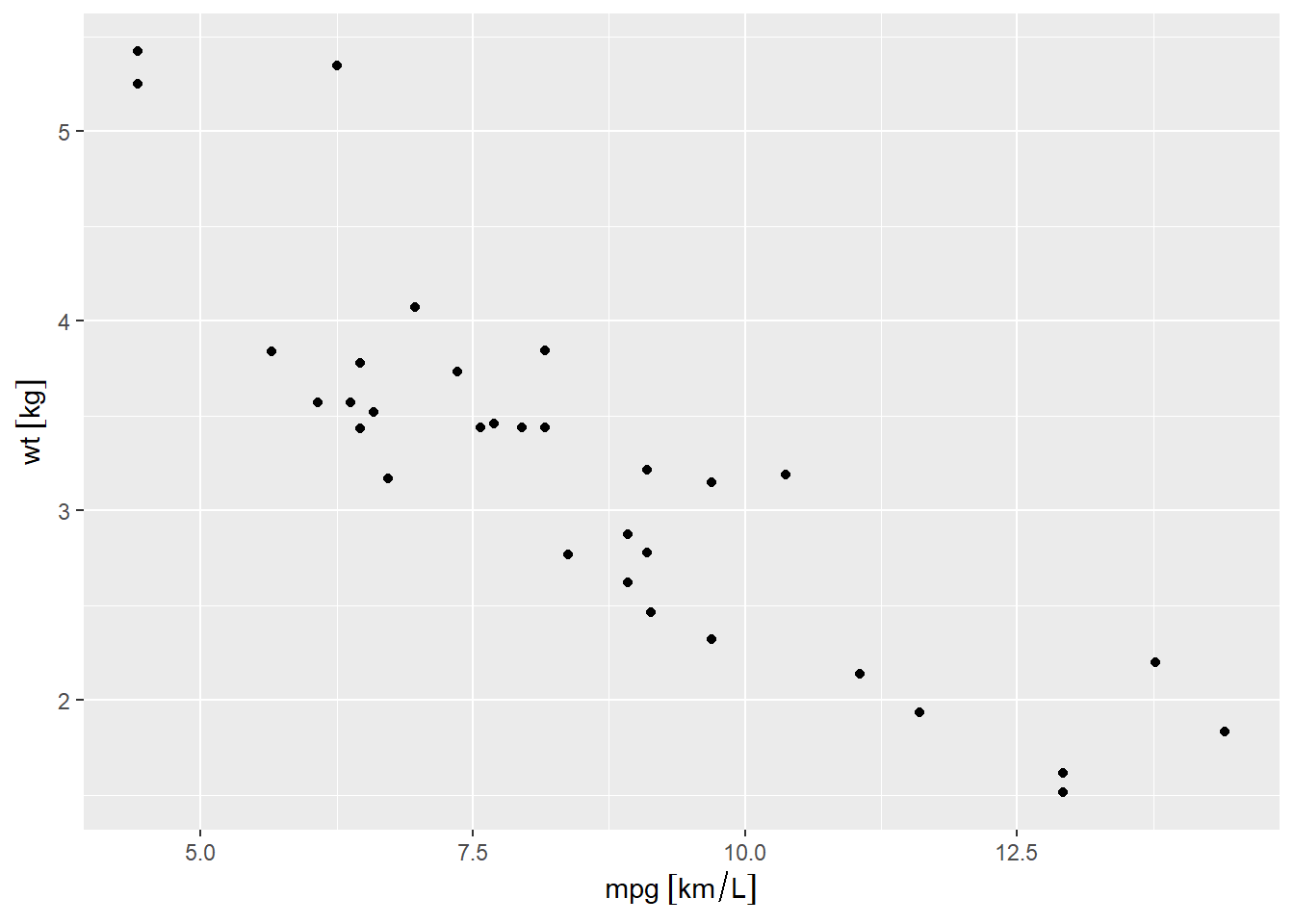Want to share your content on R-bloggers? click here if you have a blog, or here if you don't.

Data `mtcars` has a column named `mpg`. `mpg` means miles per gallon. ‘Mile’ and ‘gallon’ are units for length and volume. A mile is approximately 1.6 kilometers and a gallon is approximately 3.7 liters. Mile and gallon sound unfamiliar to people who live outside England or U.S.A. because international standard units for length and volume are meter and liter.

In this post, we will learn how to convert a unit to another unit, for instance, we will convert mpg to km/L, which is more comprehensible to people who use SI units.1

## Units in R

Vectors(the most common data structure in R) do not contain information of measurement units. Units are implicit and units should be converted by users. But as history tells us, unit conversion should be treated carefully because it can cause serious damage to the whole project2.

## Package `units`

Using the package `units`, we can easily convert units accurately. And the data is plotted, units will be included in the x- or y-label automatically.

First install package `units`.

`install.packages('units')`

And load all the necessary packages and data

```library(units)
## udunits database from C:/Users/Seul/Documents/R/win-library/4.1/units/share/udunits/udunits2.xml
library(dplyr, warn.conflicts = FALSE)
data(mtcars)```

To get the information about the data `mtcars`, we can do `help(mtcars)`. It will show the measurement unit for each column. `mpg` is measured in unit of miles per gallon, `disp` is measured in unit of cubic inch, `hp` is measured in unit of gross horsepower, `wt` is measured in unit of 1000 lbs, and `qsec` is measured in unit of sec per 1/4 mile.

It is sad that mpg(miles per gallon) is not registered in the package `units`, but we can register it ourselves. The code below installs a new unit called `mpg_US` as `interantional_mile/US_liquid_gallon`.

`install_unit(name='mpg_US', def='international_mile/US_liquid_gallon')`

Now we can use `mpg_US`. `mtcars\$mpg` is measured in unit of mpg(US) and `mtcars\$wt` is measured in unit of kilogram.

```units(mtcars\$mpg) = 'mpg_US'
units(mtcars\$wt) = 'kg'
## Units: [mpg_US]
##  21.0 21.0 22.8 21.4 18.7 18.1```

If we want to convert the unit mpg(US) to SI unit km/L, we need to do the below.3

```units(mtcars\$mpg) = 'km/L'
## Units: [km/L]
##  8.928017 8.928017 9.693276 9.098075 7.950187 7.695101```

We can easily plot the relation between `mpg` and `wt` using the package `ggplot2`. But do not forget to load `ggforce` beforehand.

```library(ggplot2)
library(ggforce) # without this, the code below will raise error!
## Registered S3 method overwritten by 'ggforce':
##   method           from
##   scale_type.units units
ggplot(data=mtcars,
aes(x=mpg, y=wt)) +
geom_point()```## Summary

• Use `units::units()<-` for setting unit for measurements.
• Use `units::units()<-` for converting unit.
• Use `units::units()<-NULL` for deleting unit.
• Use `install_unit(name=, def=)` for introducing new units.
• Use `valid_udunits()` to show all the units available from the package `units`.
```valid_udunits() %>% head
## udunits database from C:/Users/Seul/Documents/R/win-library/4.1/units/share/udunits/udunits2.xml
## # A tibble: 6 x 11
##   symbol symbol_aliases name_singular name_singular_aliases name_plural
##   <chr>  <chr>          <chr>         <chr>                 <chr>
## 1 m      ""             meter         "metre"               ""
## 2 kg     ""             kilogram      ""                    ""
## 3 s      ""             second        ""                    ""
## 4 A      ""             ampere        ""                    ""
## 5 K      ""             kelvin        ""                    ""
## 6 mol    ""             mole          ""                    ""
## # ... with 6 more variables: name_plural_aliases <chr>, def <chr>,
## #   definition <chr>, comment <chr>, dimensionless <lgl>, source_xml <chr>```

1. It is well known that the failure of MCO(Mars Climate Orbiter) is due to inadequate unit coversion.↩︎

2. If the objective is simply to reset the unit, do `units(mtcars\$mpg)=NULL; units(mtcars\$mpg)='km/L'`. This will not convert unit but just replace the unit with another unit.↩︎